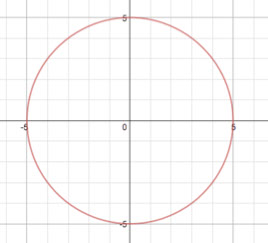# Parabolas Lesson 3 Episode 1 (Teachers)

### Making Sense

Sasha and Keoni grapple with how to represent a general point on the parabola and how to express different distances involving that point.

### Episode SupportsStudents’ Conceptual Challenges

Students might struggle to represent the distances from a point on the parabola where the y-value is general.

After struggling to label the distance from the point to the directrix, Sasha and Keoni examine their methods [see 3:01 – 4:08] to solve for the x-value of a point on the parabola when the y –value was 5, 7, and 10 to support finding a generalization. Sasha and Keoni continue to struggle with generalizing the distances in this episode.

Focus Questions

For use in a classroom, pause the video and ask these questions:

1. [Pause video at 4:08]. Keoni said it will always be y +1. What is y plus 1? Where does the one come from?

2. [Pause video at 6:03]. Keoni drew a horizontal line. What is true about every point on that line?

3. [Pause the video at 8:35]. What have Sasha and Keoni figured out so far?

Supporting Dialogue

Focus students’ attention on measurable distances and their representations by asking:

1. Where are y, y + 1, 1, and y – 1? Come up to the board and point to these distances.

2. Does anybody else see these distances represented in another place on the graph? Show us on the graph where you see them. How do you know that the distances are the same? [\bg_collapse]

Math Extensions

Consider the circle below.

1. Find the x-value of points on the circle for x-values of 3, 4, and 2.

2. Find an equation for the x-value of a point on the circle for a general y-value. Summarize your methods.